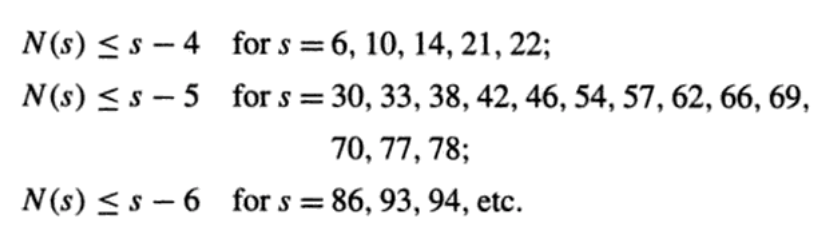# Bounding the number of orthogonal Latin squares from above

As is usual, let $$N(n)$$ denote the maximum size of a set of mutually orthogonal Latin squares of order $$n$$. I am wondering what results hold that bound $$N(n)$$ from above; the only ones I can think of are the following:

1. $$N(n)\leq n-1$$ for all $$n\geq 2$$, with equality if $$n$$ is a prime power. This is well known.

2. $$N(6)=1$$. This is also quite famous.

3. $$N(10)\leq 8$$. This was done using a computer search. (Source)

4. If $$n=1$$ or $$2~(mod~4)$$, and if $$n$$ is not a sum of two squares, then $$N(n)< n-1$$. This is the Bruck-Ryser Theorem from 1949, though stated in Latin squares instead of projective planes.

Are there any other results of this sort? I know of many results bounding $$N(n)$$ from below (mainly Beth's result that $$N(n)\geq n^{1/14.8}$$ if $$n$$ is large enough, and several results of the form "If $$n\geq n_\nu$$ then $$N(n)\geq \nu$$"), but neither I nor anyone I know can add to this list, and I haven't had much luck on Google either.